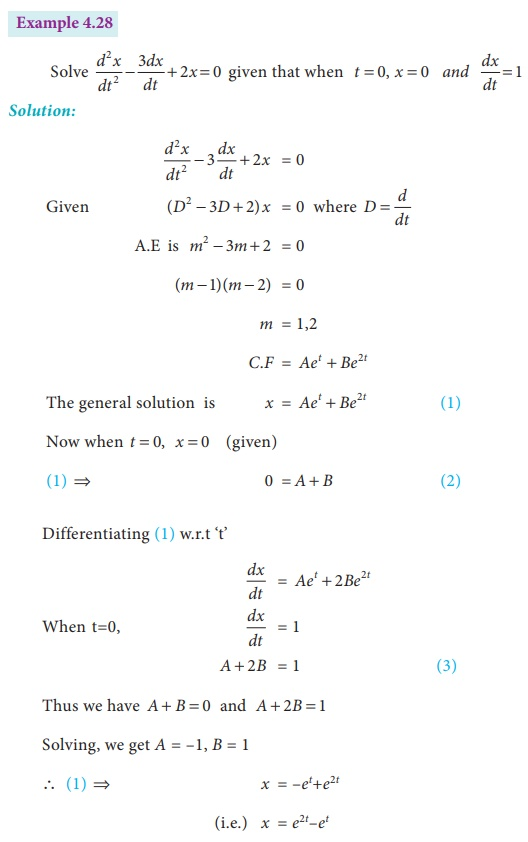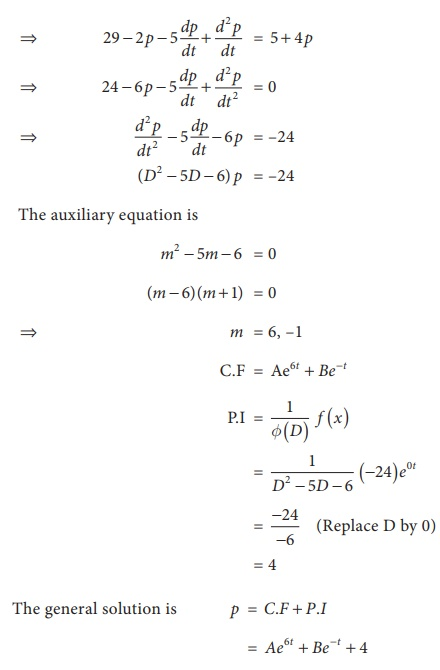Home | | Business Maths 12th Std | Second Order first degree differential equations with constant coefficients: Solved Example Problems

# Second Order first degree differential equations with constant coefficients: Solved Example Problems

Maths: Differential Equations: Second Order first degree differential equations with constant coefficients: Solved Example Problems

Example 4.25

Solve (D 2 3D 4)y = 0

Solution:

Given          (D 2 3D 4)y = 0

The auxiliary equations is

m2 3m 4 = 0

( m 4)( m + 1) = 0

m = 1, 4

Roots are real and different

The complementary function is Aex + Be4 x

The general solution is y = Aex + Be4 x

Example 4.26

Solve 9 y ′′ − 12 y′ + 4 y = 0

Solution:

Given          ( 9D2 − 12D + 4) y         = 0

The auxiliary equation is

( 3m – 2)2   = 0

(3m – 2) ( 3m – 2)          = 0    ⇒ m = 2/3,2/3

Roots are real and equal

The C.F. is ( Ax + B )e 2x/3

The general solution is y = ( Ax + B )e 2x/3Type II : f ( x ) = e ax (i.e ) f( D ) y = eaxExample 4.32

Suppose that the quantity demanded Qdand quantity supplied Qs = 5 + 4 p where p is the price. Find the equilibrium price for market clearance.

Solution:Tags : with Answer, Solution, Formula , 12th Business Maths and Statistics : Chapter 4 : Differential Equations
Study Material, Lecturing Notes, Assignment, Reference, Wiki description explanation, brief detail
12th Business Maths and Statistics : Chapter 4 : Differential Equations : Second Order first degree differential equations with constant coefficients: Solved Example Problems | with Answer, Solution, Formula